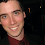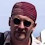## Wednesday, January 05, 2011

### Clojure: select-keys, select-values, and apply-values

Clojure provides the get and get-in functions for returning values from a map and the `select-keys` function for returning a new map of only the specified keys. Clojure doesn't provide a function that returns a list of values; however, it's very easy to create such a function (which I call `select-values`). Once you have the ability to select-values it becomes very easy to create a function that applies a function to the selected values (which I call `apply-values`).

The `select-keys` function returns a map containing only the entries of the specified keys. The following (pasted) REPL session shows a few different `select-keys` behaviors.
`user=> (select-keys {:a 1 :b 2} [:a])   {:a 1}user=> (select-keys {:a 1 :b 2} [:a :b]){:b 2, :a 1}user=> (select-keys {:a 1 :b 2} [:a :b :c]){:b 2, :a 1}user=> (select-keys {:a 1 :b 2} [])  {}user=> (select-keys {:a 1 :b 2} nil){}`
The `select-keys` function is helpful in many occassions; however, sometimes you only care about selecting the values of certain keys in a map. A simple solution is to call `select-keys` and then `vals`. Below you can find the results of applying this idea.
`user=> (def select-values (comp vals select-keys))#'user/select-valuesuser=> (select-values {:a 1 :b 2} [:a])           (1)user=> (select-values {:a 1 :b 2} [:a :b])        (2 1)user=> (select-values {:a 1 :b 2} [:a :b :c])     (2 1)user=> (select-values {:a 1 :b 2} [])             niluser=> (select-values {:a 1 :b 2} nil)nil`
The `select-values` implementation from above may be sufficient for what you are doing, but there are two things worth noticing: in cases where you might be expecting an empty list you are seeing nil; and, the values are not in the same order that the keys were specified in. Given that (standard) maps are unsorted, you can't be sure of the ordering the values.

(side-note: If you are concerned with microseconds, it's also been reported that `select-keys` is a bit slow/garbage heavy.)

An alternative definition of `select-values` uses the `reduce` function and pulls the values by key and incrementally builds the (vector) result.
`user=> (defn select-values [map ks]         (reduce #(conj %1 (map %2)) [] ks))#'user/select-valuesuser=> (select-values {:a 1 :b 2} [:a])      user=> (select-values {:a 1 :b 2} [:a :b])   [1 2]user=> (select-values {:a 1 :b 2} [:a :b :c])[1 2 nil]user=> (select-values {:a 1 :b 2} [])        []user=> (select-values {:a 1 :b 2} nil)       []`
The new `select-values` function returns the values in order and returns an empty vector in the cases where previous examples returned nil, but we have a new problem: Keys specified that don't exist in the map are now included in the vector as `nil`. This issue is easily addressed by adding a call to the `remove` function.

The implementation that includes removing nils can be found below.
`user=> (defn select-values [map ks]         (remove nil? (reduce #(conj %1 (map %2)) [] ks)))#'user/select-valuesuser=> (select-values {:a 1 :b 2} [:a])                          (1)user=> (select-values {:a 1 :b 2} [:a :b])                       (1 2)user=> (select-values {:a 1 :b 2} [:a :b :c])                    (1 2)user=> (select-values {:a 1 :b 2} [])                            ()user=> (select-values {:a 1 :b 2} nil)                           ()`
There is no "correct" implementation for `select-values`. If you don't care about ordering and nil is a reasonable return value: the first implementation is the correct choice due to it's concise definition. If you do care about ordering and performance: the second implementation might be the right choice. If you want something that follows the principle of least surprise: the third implementation is probably the right choice. You'll have to decide what's best for your context. In fact, here's a few more implementations that might be better based on your context.
`user=> (defn select-values [m ks]          (map m ks))             #'user/select-valuesuser=> (select-values {:a 1 :b 2} [:a])                                                                                     (1)user=> (select-values {:a 1 :b 2} [:a :b :c])                                                                               (1 2 nil)user=> (defn select-values [m ks]          (reduce #(if-let [v (m %2)] (conj %1 v) %1) [] ks))#'user/select-valuesuser=> (select-values {:a 1 :b 2} [:a])                                                        user=> (select-values {:a 1 :b 2} [:a :b :c])                                                  [1 2]`
Pulling values from a map is helpful, but it's generally not the end goal. If you find yourself pulling values from a map, it's likely that you're going to want to apply a function to the extracted values. With that in mind, I generally define an `apply-values` function that returns the result of applying a function to the values returned from specified keys.

A good example of this is returning the total for a line item represented as a map. Given a map that specifies a line item costing \$5 and having a quantity of 4, you can use `(* price quantity)` to determine the total price for the line item.

Using our previously defined `select-values` function we can do the work ourselves, as the example below shows.
`user=> (let [[price quantity] (select-values {:price 5 :quantity 4 :upc 1123} [:price :quantity])]                                   (* price quantity))20`
The example above works perfectly well; however, applying a function to the values of a map seems like a fairly generic operation that can easily be extracted to it's own function (the `apply-values` function). The example below shows the definition and usage of my definition of `apply-values`.
`user=> (defn apply-values [map f & ks]                   (apply f (select-values map ks)))#'user/apply-valuesuser=> (apply-values {:price 5 :quantity 4 :upc 1123} * :price :quantity)20`
I find select-keys, select-values, & apply-values to be helpful when writing Clojure applications. If you find you need these functions, feel free to use them in your own code. However, you'll probably want to check the comments - I'm sure someone with more Clojure experience than I have will provide superior implementations.

1.Looks like there is a typo in this definition of of select-values

(defn select-values [m ks]
(map #({:a 1 :b 2} %) ks))

Probably was supposed to be:

(defn select-values [m ks]
(map #(m %) ks))

2.Hello

Could you add clojure tag to your post, so it will appear in Planet Clojure?

3.Anonymous8:35 AM

@Jake, yes, it is a typo. Thanks, I've updates the example.

@Alex: done.

4.There's also map destructuring:

(let [{:keys [price quantity]} {:price 5 :quantity 4 :upc 1123}] (* price quantity))

5.Anonymous11:59 PM

apply-values is an interesting name.

apply takes function as first arg, apply-values does not, it goes for the map function style of map as the first arg.

Also apply takes the args as a vector but apply-values does not, so you'd end up having to do (apply apply-values ...) when you have a collection of keys.

For one offs Brian's destructing code is what I first though of, but I rather like (apply * ((juxt :price :quantity) m)) too.

6.jurgen11:25 AM

typo:
(defn select-values [m ks]
(reduce #(if-let [v ({:a 1 :b 2} %2)] (conj %1 v) %1) [] ks))

should be:
(defn select-values [m ks]
(reduce #(if-let [v (m %2)] (conj %1 v) %1) [] ks))

7.Anonymous11:48 AM

thanks jurgan. updated.